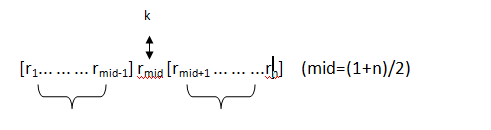# python 标准库 bisect 二分查找## 何谓二分查找## 对比顺序查找python标准库中已经实现了二分查找。

## 函数

• `bisect.bisect_left`(a, x, lo=0, hi=len(a))

a 中找到 x 合适的插入点以维持有序。参数 lohi 可以被用于确定需要考虑的子集；默认情况下整个列表都会被使用。如果 x 已经在 a 里存在，那么插入点会在已存在元素之前（也就是左边）。如果 a 是列表（list）的话，返回值是可以被放在 `list.insert()` 的第一个参数的。

• `bisect.bisect_right`(a, x, lo=0, hi=len(a))

• `bisect.bisect`(a, x, lo=0, hi=len(a))

类似于 `bisect_left()`，但是返回的插入点是 a 中已存在元素 x 的右侧。

返回的插入点 i 可以将数组 a 分成两部分。左侧是 `all(val <= x for val in a[lo:i])`，右侧是 `all(val > x for val in a[i:hi])` for the right side。

• `bisect.insort_left`(a, x, lo=0, hi=len(a))

x 插入到一个有序序列 a 里，并维持其有序。如果 a 有序的话，这相当于 `a.insert(bisect.bisect_left(a, x, lo, hi), x)`。要注意搜索是 O(log n) 的，插入却是 O(n) 的。

• `bisect.insort_right`(a, x, lo=0, hi=len(a))

• `bisect.insort`(a, x, lo=0, hi=len(a))

类似于 `insort_left()`，但是把 x 插入到 a 中已存在元素 x 的右侧。

SortedCollection recipe 使用 bisect 构造了一个功能完整的集合类，提供了直接的搜索方法和对用于搜索的 key 方法的支持。所有用于搜索的键都是预先计算的，以避免在搜索时对 key 方法的不必要调用。

## 其他示例

`sorted()` 函数不同，对于 `bisect()` 函数来说，key 或者 reversed 参数并没有什么意义。因为这会导致设计效率低下（连续调用 bisect 函数时，是不会 “记住” 过去查找过的键的）。